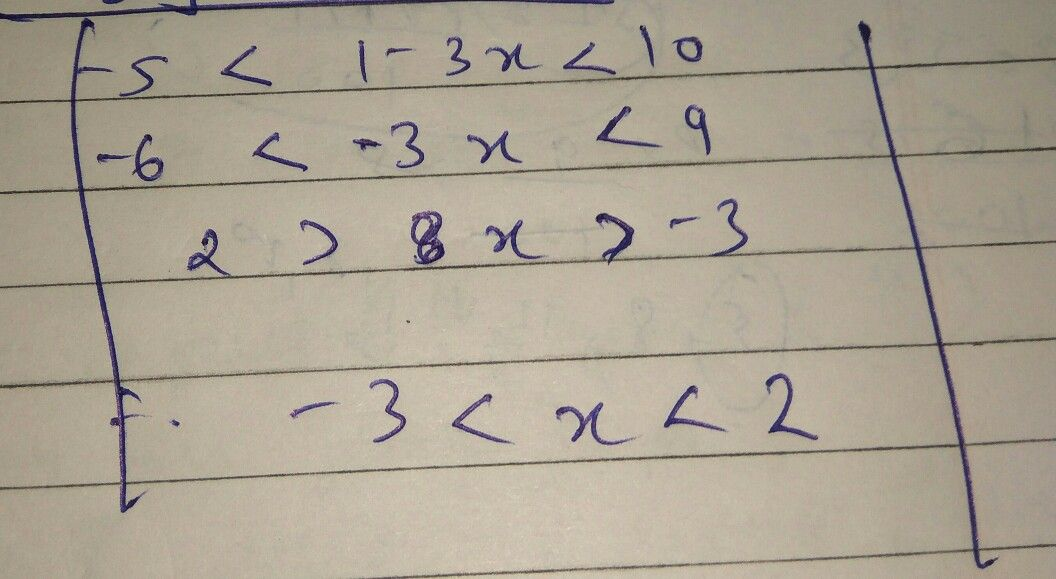Symbol
Problem$+19$ Which of the following is the solution statement for the inequality shown below? $-5<1-$ $3x<10$ F. G. $-5<x<1$ $-3<x$ $-3<x<2$ H. J. $-2<$ $x<3$ K. $x<-30rx>2$
Algebra
SolutionQanda teacher - Vishal
wait